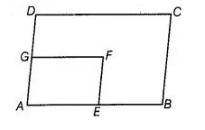# In figure, ABCD and AEFG are`
Question:

In figure, ABCD and AEFG are two parallelograms. If ∠C = 55°, then determine ∠F.Solution:

We have, ABCD and AEFG are two parallelograms and ∠C = 55°. Since, ABCD is a parallelogram, then opposite angles of a parallelogram are

equal.

∠A = ∠C = 55° ...(i)

Also, AEFG is a parallelogram.

∴ ∠A=∠F = 55° [from Eq. (i)]